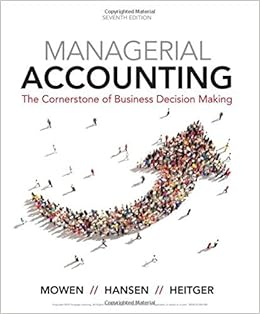# Refer to table 12 1 suppose the fixed cost of

• Test Prep
• 11
• 95% (263) 250 out of 263 people found this document helpful

This preview shows page 2 - 5 out of 11 pages.

##### We have textbook solutions for you!
The document you are viewing contains questions related to this textbook.The document you are viewing contains questions related to this textbook.
Chapter 7 / Exercise 7-32
Managerial Accounting: The Cornerstone of Business Decision-Making
Hansen/MowenExpert Verified
8) Refer to Table 12-1. Suppose the fixed cost of production rises by \$500 and the price per unit is still \$8. What happens to the firm's profit-maximizing output level? A) It must fall. B) It must rise to offset the increased cost. C) It will remain the same. D) The firm will shut down. Answer: C
9) Refer to Table 12-1. The firm will not produce in the short run if the output price falls below D
Figure 12-1
##### We have textbook solutions for you!
The document you are viewing contains questions related to this textbook.The document you are viewing contains questions related to this textbook.
Chapter 7 / Exercise 7-32
Managerial Accounting: The Cornerstone of Business Decision-Making
Hansen/MowenExpert Verified
10) Refer to Figure 12-1. If the firm is producing 700 units C
11) Refer to Figure 12-1. If the firm is producing 700 units, what is the amount of its profit or loss? A A D
12) Refer to Figure 12-1. If the firm is producing 200 units A) it breaks even. B) it is making a loss. C) it should cut back its output to maximize profit. D) it should increase its output to maximize profit. Answer: D
14) Refer to Figure 12-3. If the firm is producing 500 units, what is the amount of its profit or loss? A A D
18) If, for a perfectly competitive firm, price exceeds the marginal cost of production, the firm should A
Figure 12-2
19) Refer to Figure 12-2. What is the amount of profit if the firm produces Q 2 units? g . 2 . 2 . . A
20) Refer to Figure 12-2. Suppose the firm is currently producing Q 2 units. What happens if it expands output to Q 3 units? A) Its profit increases by the size of the vertical distance df B) It makes less profit. C) It incurs a loss. D) It will be moving toward its profit maximizing output. Answer: . B
12.3 Illustrating Profit or Loss on the Cost Curve Graph 1) A firm's total profit can be calculated as all of the following except D
•••